##### Venture Capital For Dummies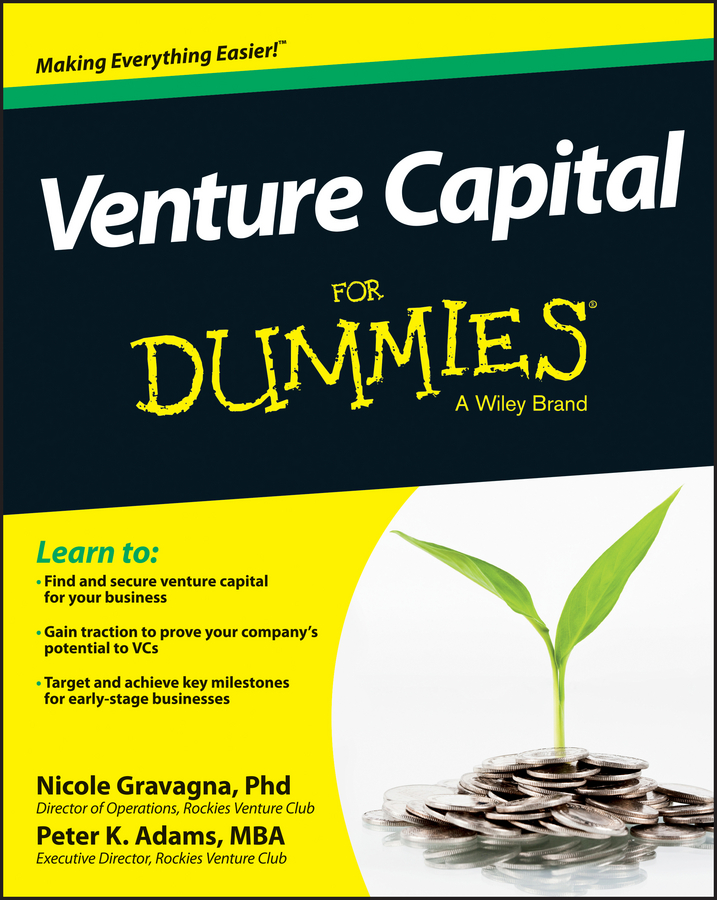The cost of using external funds, or the cost of debt capital, is the interest rate you must pay lenders. However, because interest expenses are tax deductible, the after-tax cost of debt, kd, is the interest rate, r, multiplied by 1 minus the firm’s marginal tax rate, t, or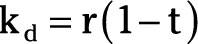You’ve decided to finance a capital investment by issuing bonds that have a 6 percent annual interest rate. In addition, your company’s marginal tax rate is 35 percent. The cost of using debt to finance the project equals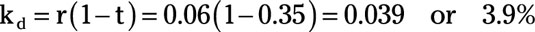Firms commonly raise funds for capital investment from both internal and external sources. The composite cost of capital, kc, is a weighted average of the cost of equity capital and the cost of debt capital. Therefore,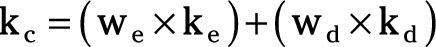where we and wd are the weights or proportions of equity and debt capital you use to finance the project.

You decide to fund a capital investment through a combination of equity and debt capital. You plan to fund 25 percent of your capital investment through equity and 75 percent through debt.

To determine the composite cost of capital, start by separately calculating the cost of equity capital and the cost of debt capital. Assume your firm’s â coefficient is 2.1 and the average stock return is 6 percent. The return on U.S. Treasury bills is 1 percent, which is the risk-free rate of return. Your firm can borrow funds at 9-percent interest and its marginal tax rate is 34 percent.

1. Determine the cost of equity capital.

This example uses the capital-asset-pricing method.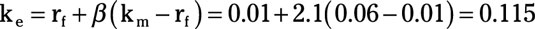2. Determine the cost of debt by using the marginal tax rate.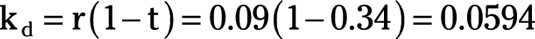3. Determine the composite cost of capital.

Remember to weight each component by the percentage of funds raised through that method — equity or debt.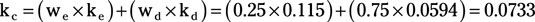Thus, the composite cost of capital is 7.33 percent.